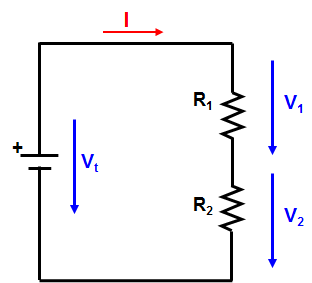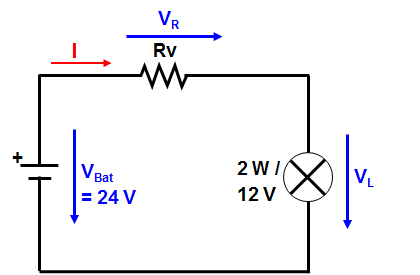Series connection of Resistors

### Series connection of Resistors

The following applies in general for series connection:

The same current flows through each part of a series circuit.
There is only one current

Voltage applied to a series circuit is equal to the sum of the individual voltage drops.

Vt =  V1  +  V2 + … +  Vn         (1)

The total resistance of a series circuit is equal to the sum of individual resistances.

R = R1 + R2 + … + Rn            (2)

We proof this:

Eq. (1) divided by I:

Vt =   V1  +  V2 + … +  Vn
.I         I         I                I

=>    According to the Law of Ohm we get equation (2).

Work order:   Calculate the necessary series resistor!Work order - Series Resistor